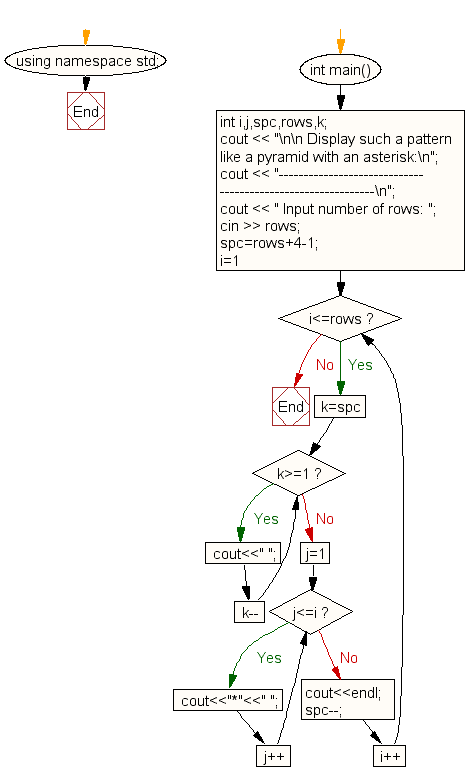﻿ C++ : Show a pattern like a pyramid with an asterisk

# C++ Exercises: Display the pattern like a pyramid with an asterisk

## C++ For Loop: Exercise-40 with Solution

Write a C++ program to make such a pattern like a pyramid with an asterisk.

Sample Solution:

C++ Code :

``````#include <iostream>
#include <string>
using namespace std;

int main()
{
int i,j,spc,rows,k;
cout << "\n\n Display such a pattern like a pyramid with an asterisk:\n";
cout << "------------------------------------------------------------\n";
cout << " Input number of rows: ";
cin >> rows;
spc=rows+4-1;
for(i=1;i<=rows;i++)
{
for(k=spc;k>=1;k--)
{
cout<<" ";
}
for(j=1;j<=i;j++)
cout<<"*"<<" ";
cout<<endl;
spc--;
}
}
``````

Sample Output:

``` Display such a pattern like a pyramid with an asterisk:
------------------------------------------------------------
Input number of rows: 5
*
* *
* * *
* * * *
* * * * *
```

Flowchart:C++ Code Editor: